# EXAMPLES  - TYPES - HYBRIDIZATION IN CHEMISTRY

Almost always, some sort of intermixing i.e., hybridization of pure atomic orbitals is observed before the bond formation to confer maximum stability to the molecule. On this page, examples of different types of hybridization in chemistry are discussed with illustrations.

If you are not sure .....What is Hybridization in chemistry?....Watch the following video

## sp HYBRIDIZATION - EXAMPLE

### 1) Beryllium Chloride (BeCl2)

* The electronic configuration of 'Be' in ground state is 1s2 2s2. Since there are no unpaired electrons, it undergoes excitation by promoting one of its 2s electron into empty 2p orbital.

Thus in the excited state, the electronic configuration of Be is 1s2 2s1 2p1.

If the beryllium atom forms bonds using these pure orbitals, the molecule might be angular. However the observed shape of BeCl2 is linear. To account for this, sp hybridization was proposed as explained below.

* In the excited state, the beryllium atom undergoes 'sp' hybridization by mixing a 2s and one 2p orbitals. Thus two half filled 'sp' hybrid orbitals are formed, which are arranged linearly.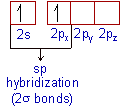* These half filled sp-orbitals form two σ bonds with two 'Cl' atoms.

* Thus BeCl2 is linear in shape with the bond angle of 180o.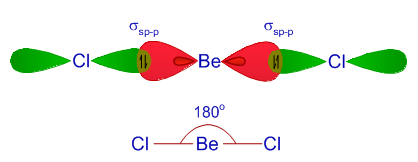### 2) Acetylene (C2H2)

* The ground state electronic configuration of 'C' is 1s2 2s2 2px12py1. There are only two unpaired electrons in the ground state. However, the valency of carbon is four i.e., it forms 4 bonds. In order to form four bonds, there must be four unpaired electrons. Hence carbon promotes one of its 2s electron into the empty 2pz orbital in the excited state.

Thus in the excited state, the electronic configuration  of carbon is 1s2 2s1 2px12py12pz1* Each carbon atom undergoes 'sp' hybridization by using a 2s and one 2p orbitals in the excited state to give two half filled 'sp' orbitals, which are arranged linearly.

* The two carbon atoms form a σsp-sp bond with each other by using sp-orbitals.

However there are also two unhybridized p orbitals i.e., 2py and 2pz on each carbon atom which are perpendicular to the sp hybrid orbitals. These orbitals form two πp-p bonds between the two carbon atoms.

Thus a triple bond (including one σsp-sp bond & two πp-p bonds ) is formed between carbon atoms.

* Each carbon also forms a σsp-s bond with the hydrogen atom.

* Thus acetylene molecule is linear with 180o of bond angle.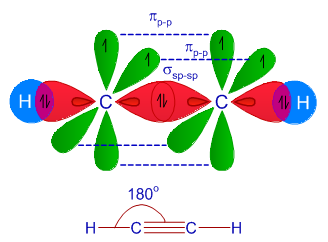## sp2 HYBRIDIZATION - EXAMPLE

### 1) Boron trichloride (BCl3)

* The electronic configuration of 'B' in ground state is 1s2 2s2 2p1 with only one unpaired electron. Since the formation of three bonds with chlorine atoms require three unpaired electrons, there is promotion of one of 2s electron into the 2p sublevel by absorbing energy.

Thus Boron atom gets electronic configuration: 1s2 2s2 2px12py1

However to account for the trigonal planar shape of this BCl3 molecule, sp2 hybridization before bond formation was put forwarded.

* In the excited state, Boron undergoes sp2 hybridization by using a 2s and two 2p orbitals to give three half filled sp2 hybrid orbitals which are oriented in trigonal planar symmetry.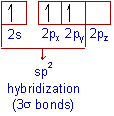* Boron forms three σsp-p bonds with three chlorine atoms by using its half filled sp2 hybrid orbitals. Each chlorine atom uses it's half filled p-orbital for the σ-bond formation.

* Thus the shape of BCl3 is trigonal planar with bond angles equal to 120o.### 2) Ethylene (C2H4)

* During the formation of ethylene molecule, each carbon atom undergoes sp2 hybridization in its excited state by mixing 2s and two 2p orbitals to give three half filled sp2 hybrid orbitals oriented in trigonal planar symmetry.

There is also one half filled unhybridized 2pz orbital on each carbon perpedicular to the plane of sp2 hybrid orbitals.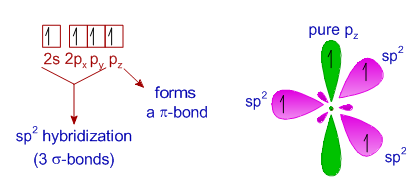* The carbon atoms form a σsp2-sp2 bond with each other by using sp2 hybrid orbitals.

A πp-p bond is also formed between them due to lateral overlapping of unhybridized 2pz orbitals.

Thus there is a double bond (σsp2-sp2πp-p) between two carbon atoms.

* Each carbon atom also forms two σsp2-s bonds with two hydrogen atoms.

* Thus ethylene molecule is planar with ∠HCH & ∠HCC bond angles equal to 120o

* All the atoms are present in one plane.## sp3 HYBRIDIZATION - EXAMPLE

### 1) Methane (CH4)

* During the formation of methane molecule, the carbon atom undergoes sp3 hybridization in the excited state by mixing one ‘2s’ and three 2p orbitals to furnish four half filled sp3 hybrid orbitals, which are oriented in tetrahedral symmetry in space around the carbon atom.

* Each of these sp3 hybrid orbitals forms a σsp3-s bond with one hydrogen atom. Thus carbon forms four σsp3-s bonds with four hydrogen atoms.

* Methane molecule is tetrahedral in shape with 109o28' bond angle.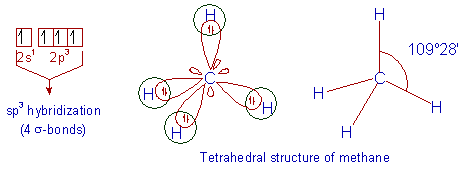### 2) Ethane (C2H6)

* Just like in methane molecule, each carbon atom undergoes sp3 hybridization in the excited state to give four sp3 hybrid orbitals in tetrahedral geometry.

* The two carbon atoms form a σsp3-sp3 bond with each other due to overlapping of sp3 hybrid orbitals along the inter-nuclear axis.

Each carbon atom also forms three σsp3-s bonds with hydrogen atoms.

* Thus there is tetrahedral symmetry around each carbon with ∠HCH & ∠HCC bond angles equal to 109o28'.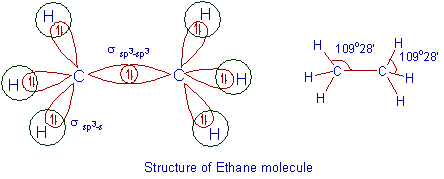### 3) Ammonia (NH3)

* The ground state electronic configuration of nitrogen atom is: 1s2 2s2 2px12py12pz1. Since there are three unpaired electrons in the 2p sublevel, the nitrogen atom can form three bonds with three hydrogen atoms. This will give ammonia molecule with 90o of bond angles. However, the bond angles are reported to be 107o48'.

* Therefore, it was proposed that, the Nitrogen atom undergoes sp3 hybridization of a 2s and three 2p orbitals to give four sp3 orbitals, which are arranged in tetrahedral symmetry. It is clear that this arrangement will give more stability to the molecule due to minimization of repulsions.

Among them three are half filled and one is full filled.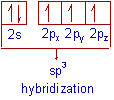* Nitrogen atom forms 3 σsp3-s bonds with three hydrogen atoms by using three half filled sp3 hybrid orbitals. There is also a lone pair on nitrogen atom belonging to the full filled sp3 hybrid orbital. It occupied more space than the bond pairs.

* However, the ∠HNH bond angle is not equal to normal tetrahedral angle: 109o28'. The reported bond angle is 107o48'. The observed decrease in the bond angle is due to the repulsion caused by lone pair over the bond pairs.

That is why, ammonia molecule is trigonal pyramidal in shape with a lone pair on nitrogen atom.### 4) Water molecule (H2O)

* The electronic configuration of oxygen is 1s2 2s2 2px22py12pz1. There are two unpaired electrons in oxygen atom, which may form bonds with hydrogen atoms. However the the bond angles in the resulting molecule should be equal to 90o.

The experimental bond angles reported were equal to 104o28'. To account this, sp3 hybridization before the bond formation was proposed.

* During the formation of water molecule, the oxygen atom undergoes sp3 hybridization by mixing a 2s and three 2p orbitals to furnish four sp3 hybrid orbitals oriented in tetrahedral geometry.

Among them,  two are half filled and the remaining two are completely filled.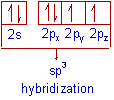* Now the oxygen atom forms two σsp3-s bonds with hydrogen atoms by using half filled hybrid orbitals.

* The  reported bond angle is 104o28' instead of regular tetrahedral angle: 109o28'. It is again due to repulsions caused by two lone pairs on the bond pairs.

Thus water molecule gets angular shape (V shape).## sp3d HYBRIDIZATION - EXAMPLE

### 1) Phosphorus pentachloride(PCl5)

* The ground state electronic configuration of phosphorus atom is: 1s2 2s22p6 3s23px13py13pz1

* The formation of PCl5 molecule requires 5 unpaired electrons. Hence the phosphorus atom undergoes excitation to promote one electron from 3s orbital to one of empty 3d orbital.

* Thus the electronic configuration of 'P' in the excited state is 1s2 2s22p6 3s13px13py13pz1 3d1.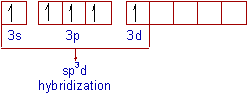* In the excited state, intermixing of a 3s, three 3p and one 3d orbitals to give five half filled sp3d hybrid orbitals, which are arranged in trigonal bipyramidal symmetry.

i.e., Three orbitals are arranged in trigonal planar symmetry, whereas the remaining two are arranged perpendicularly above and below this plane.

* By using these half filled sp3d orbitals, phosphorous forms five σsp3d-p bonds with chlorine atoms. Each chlorine atom makes use of half filled 3pz orbital for the bond formation.

* The shape of PCl5 molecule is trigonal bipyramidal with 120o and 90o of ∠Cl - P - Cl bond angles.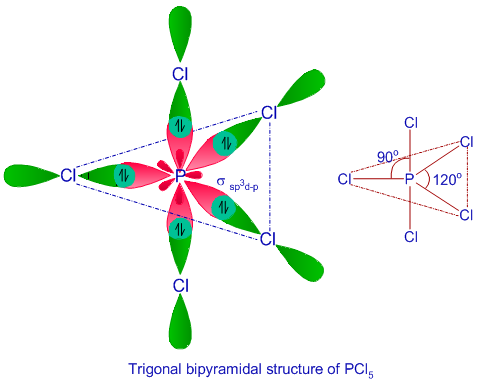## sp3d2 HYBRIDIZATION - EXAMPLE

### 1) Sulfur hexaflouride (SF6)

* The electronic configuration of 'S' in ground state is 1s2 2s22p6 3s23px23py13pz1.

* In SF6 molecule, there are six bonds formed by sulfur atom. Hence there must be 6 unpaired electrons. However there are only 2 unpaired electrons in the ground state of sulfur. Hence it promotes two electrons into two of the 3d orbitals (one from 3s and one from 3px).

* Thus the electronic configuration of 'S' in its 2nd excited state is 1s2 2s22p6 3s13px13py13pz13d2.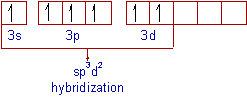* In the second excited state, sulfur under goes sp3d2 hybridization by mixing a 3s, three 3p and two 3d orbitals. Thus formed six half filled sp3d2 hybrid orbitals are arranged in octahedral symmetry.

Sulfur atom forms six σsp3d2-p bonds with 6 fluorine atoms by using these sp3d2 orbitals. Each fluorine atom uses is half-filled 2pz orbitals for the bond formation. SF6 is octahedral in shape with bond angles equal to 90o.## sp3d3 HYBRIDIZATION - EXAMPLE

### 1) Iodine heptafluoride (IF7):

* The electronic configuration of Iodine atom in the ground state is: [Kr]4d105s25p5. Since the formation of IF7 requires 7 unpaired electrons, the iodine atom promotes three of its electrons (one from 5s orbital and two from 5p sublevel) into empty 5d orbitals. This state is referred to as third excited state.

* The electronic configuration of Iodine in the third excited state can be written as: [Kr]4d105s15p35d3.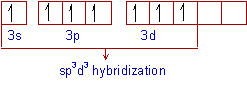In the third excited state, iodine atom undergoes sp3d3 hybridization to give 7 half filled sp3d3 hybrid orbitals in pentagonal bipyramidal symmetry. These will form 7 σsp3d3-p bonds with fluorine atoms.

Thus the shape of IF7 is pentagonal bipyramidal. The ∠F-I-F bond angles in the pentagonal plane are equal to 72o, whereas two fluorine are present perpendicularly to the pentagonal plane above and below by making 90o of bond angle with the I-F bonds on pentagonal plane.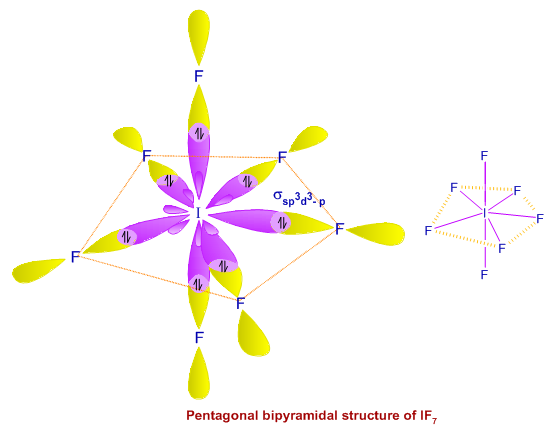## Hybridization Questions & Answers (MCQ)

Question-1) Which of the following are examples for sp hybridisation?

A) Acetylene

B) Boron trifluoride

C) Beryllium chloride

D) Carbon dioxide

E) Ethylene

Question-2) Which of the following is example of sp3d hybridization?

A) IF7

B) BCl3

C) CH4

D) PCl5

Question-3) In the carbonyl group, hybridization of C atom is:

(a) sp

(b) sp2

(c) sp3

(d) sp3

Question-4) What are the bond angles of molecules showing sp3d hybridization in the central atom?

(a) 180o and 120o

(b) 90o and 180o

(c) 90o and 120o

(d) 120o and 100o

Around the sp3d central atom, the bond angles are 90o and 120o.

Question-5) What is the hybridization in BF3 molecule?

(a) sp

(b) sp2

(c) sp3

(d) sp3

Question-6) What is the bond angle in beryllium chloride molecule?

(a) 180o

(b) 90o

(c) 120o

(d) 109o28'

Question-7) What is the shape of IF7 molecule?

1) Tetrahedral

2) Square planar

3) Octahedral

4) Pentagonal bipyramidal

Question-8) What is the hybridization in BeCl2?

(a) sp hybridization

(b) sp2 hybridization

(c) sp3 hybridization

(d) sp3d hybridization

Question-9) What is the shape of methane molecule?

1) Tetrahedral

2) Square planar

3) Octahedral

4) Pentagonal bipyramidal

Question - 10) What are the bond angles in PCl5 molecule?

(a) 120o and 109o28'

(b) 90o and 180o

(c) 90o and 120o

(d) 180o and 120o

Question - 11) In which excited state iodine shows sp3d3 hybridization

(a) 2nd

(b) 3rd

(c) 4th

(d) 1st

Question - 12) What shapes are associated with sp3d and sp3d2 hybrid orbitals, respectively?

1) Tetrahedral & linear

2) Pentagonal bipyramidal & octahedral

3) Octahedral & pentagonal bipyramidal

4) Pentagonal bipyramidal & Tetrahedral

Question - 13) What atomic or hybrid orbitals make up the sigma bond between Be and Cl in beryllium chloride, BeCl2?

1) sp2 & p

2) sp3 & s

3) sp & s

4) sp & p

Question - 14) In the carbonyl group, hybridization of C atom is:

1) sp

2) sp2

3) sp3

4) sp3d